Courses

# Test: Data Handling- 3

## 20 Questions MCQ Test Mathematics (Maths) Class 8 | Test: Data Handling- 3

Description
This mock test of Test: Data Handling- 3 for Class 8 helps you for every Class 8 entrance exam. This contains 20 Multiple Choice Questions for Class 8 Test: Data Handling- 3 (mcq) to study with solutions a complete question bank. The solved questions answers in this Test: Data Handling- 3 quiz give you a good mix of easy questions and tough questions. Class 8 students definitely take this Test: Data Handling- 3 exercise for a better result in the exam. You can find other Test: Data Handling- 3 extra questions, long questions & short questions for Class 8 on EduRev as well by searching above.
QUESTION: 1

Solution:
QUESTION: 2

Solution:
QUESTION: 3

### Frequency distribution of daily income of 550 workers of a factory is given bellow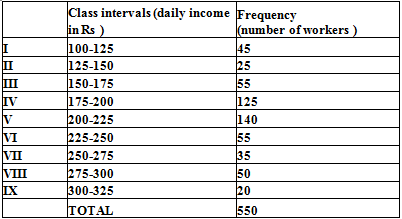Q. Which class has the lowest frequency ?

Solution:
QUESTION: 4

Frequency distribution of daily income of 550 workers of a factory is given bellowQ. What is the upper limit of the class interval 250-275?

Solution:
QUESTION: 5

Frequency distribution of daily income of 550 workers of a factory is given bellowQ. Which two classes have the same frequency?

Solution:

The classes (150–175) and (225-250) are having the same frequency (which is 55).

QUESTION: 6

The number of hours for which students of particular class watched television during holidays is shown through the graph given below: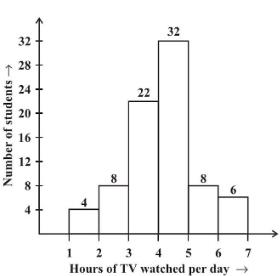Q. For how many hours did the maximum number of students watch TV?

Solution:

From the graph, it can be observed that the maximum number of students (i.e., 32) watched TV for 4 − 5 hours.

QUESTION: 7

The number of hours for which students of particular class watched television during holidays is shown through the graph given below: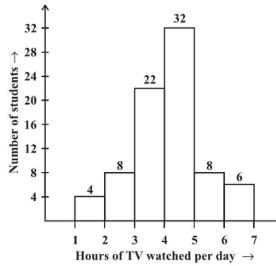Q. How many students watched TV for less than 4 hrs ?

Solution:

Number of students watching TV for less than 4 hours = 4 + 8 + 22 = 34

QUESTION: 8

The number of hours for which students of particular class watched television during holidays is shown through the graph given below:Q. How many students spent more than 5 hrs in TV watching ?

Solution:

14 students spent more than 5 hours in watching TV.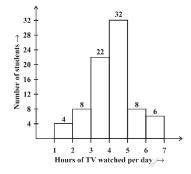The number of students who watched TV for >5 hours =  number of students who watched TV for (5 – 6 hours) + (6 – 7 hours).

So the no. of students = 8 + 6 = 14

QUESTION: 9

The number of hours for which students of particular class watched television during holidays is shown through the graph given below:Q. For how many hours did the minimum number of students watch TV ?

Solution:
QUESTION: 10

The number of hours for which students of particular class watched television during holidays is shown through the graph given below:Q. How many students spent less than 5 hrs in TV watching ?

Solution:
QUESTION: 11

Which of the following is the probability of an impossible event?

Solution:
QUESTION: 12

Adjoining pie-chart gives the expenditure (in %age) on various items and savings of a family during a month .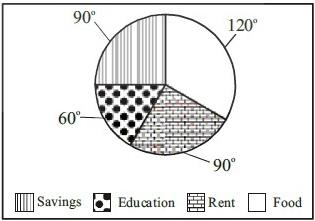Q. On which item the expenditure was minimum ?

Solution:
QUESTION: 13

A coin is tossed. Which of the following is the probability of getting a head or tail?

Solution: The probability of getting heads or tails when a coin is tossed is 1 Result 0-neither tails nor heads 1/2-1/2heads and 1/2tails.
QUESTION: 14

Adjoining pie-chart gives the expenditure (in %age) on various items and savings of a family during a month .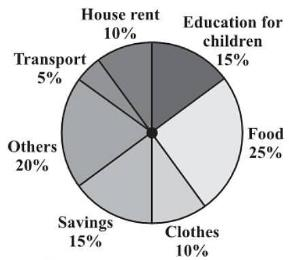Q. If the monthly savings of the family is Rs 3000, What is the monthly expenditure on cloths ?

Solution:

Detailed solution not provided.
Ans. (i) Transport
(ii) Education for children
(iii) Rs 2000

QUESTION: 15

The range of the data: 6,14,20,16,6,5,4,18,25,15, and 5 is

Solution:

The option which is choosed is correct because

range=Highest observation -  Lowest observation.

so here in the given question highest observation is 25 and the lowest observation is 4 if we subtract it we will get 21

QUESTION: 16

Numbers 1 to 10 are written on ten separates slips (one number on one slip), kept in a box and mixed well. One slip is chosen from the box without looking in to it .

Q. What is the probability of getting a number 6?

Solution:
QUESTION: 17

Numbers 1 to 10 are written on ten separates slips (one number on one slip), kept in a box and mixed well. One slip is chosen from the box without looking in to it.

Q. What is the probability of getting a number less than 6?

Solution:
QUESTION: 18

Numbers 1 to 10 are written on ten separates slips (one number on one slip), kept in a box and mixed well. One slip is chosen from the box without looking in to it.

Q. What is the probability of getting a number greater than 6?

Solution:
QUESTION: 19

Numbers 1 to 10 are written on ten separates slips (one number on one slip), kept in a box and mixed well. One slip is chosen from the box without looking in to it.

Q. What is the probability of getting a 1-digit number ?

Solution: There are 9 one digit numbers out of 10 numbers ( 1 to 10 ). So probability of getting a one digit number is 9/10
QUESTION: 20

Numbers 1 to 10 are written on ten separates slips (one number on one slip), kept in a box and mixed well. One slip is chosen from the box without looking in to it.

Q. What is the probability of getting an even number ?

Solution:

Probability of even number =No. of favourable outcomes/ total outcomes= 5/10=1/2